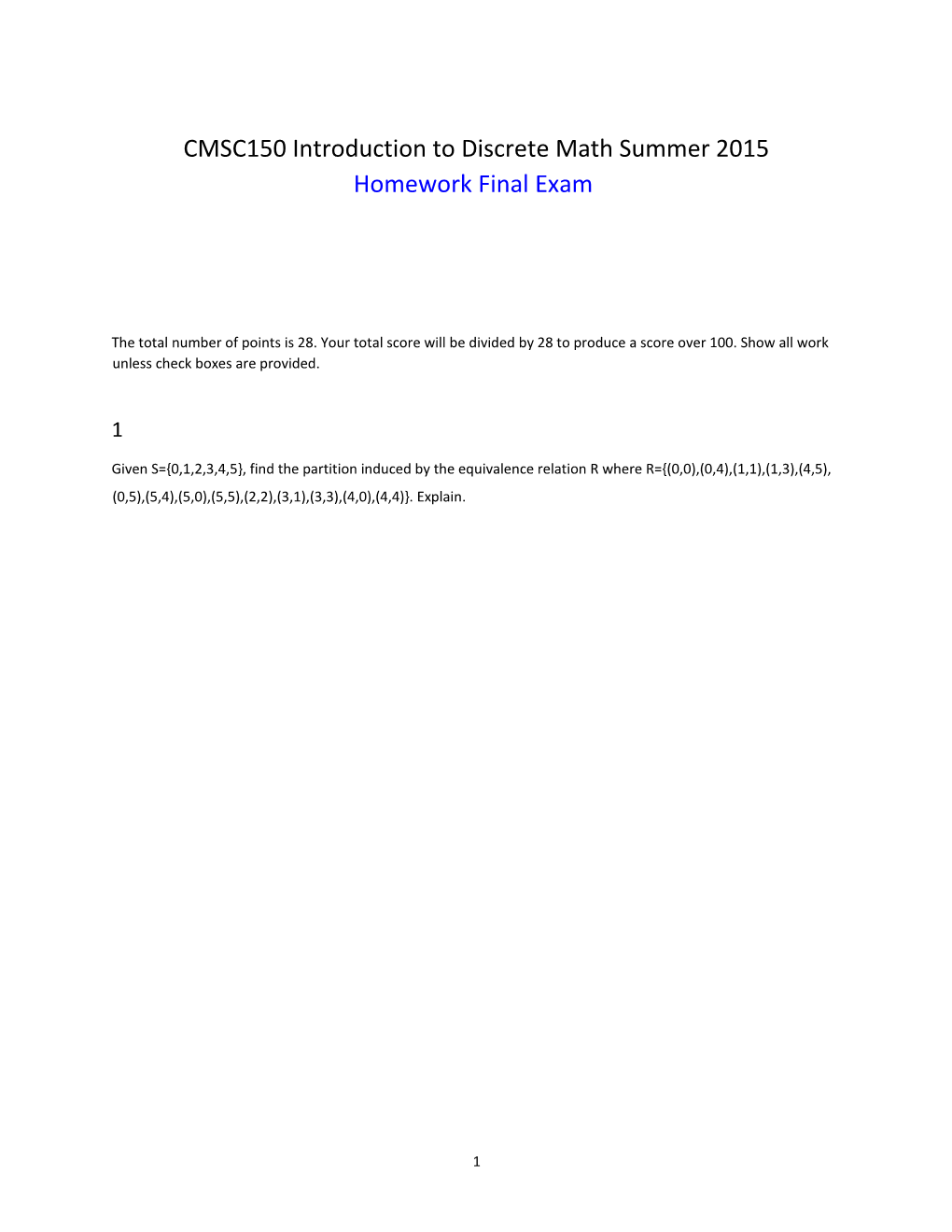# CMSC150 Introduction to Discrete Math Summer 2015## CMSC150 Introduction to Discrete Math Summer 2015

Homework Final Exam

The total number of points is 28. Your total score will be divided by 28 to produce a score over 100. Show all work unless check boxes are provided.

1

Given S={0,1,2,3,4,5}, find the partition induced by the equivalence relation R where R={(0,0),(0,4),(1,1),(1,3),(4,5),(0,5),(5,4),(5,0),(5,5),(2,2),(3,1),(3,3),(4,0),(4,4)}. Explain.

2

Let A and B be any sets. Prove the following set identity using the laws of set theory (set identities). Justify each step with the law you used. Missing steps and missing justification will be penalized. (1 point)

A ∩(B ∪A’) ∩B’ = Ø

3

Let the relation R = {(0,0), (0,3), (1,0), (1,2), (2,0), (3,2)}

Find R’ the transitive closure of R. (1 point)

4

Using the predicate symbols shown and appropriate quantifiers, write each English language statement as a predicate wff. (The domain is the whole world.)

B(x) is “x is a ball.”

R(x) is “x is round.” S(x) is “x is a soccer ball.”

1.All balls are round.

2.Not all balls are soccer balls.

3.All soccer balls are round.

4.Some balls are not round.

5

Do a proof by mathematical induction using the 4 steps below to show that the following statement is true for every positive integer n:

Hint: Use the weak principle of mathematical induction

2+6+18+...+2.3n−1 = 3n−1

Note: 2.3n−1is not the decimal 2.3 to the n-1 power but 2 times 3 to the n-1 power.

1.Prove the base step (1 point).

2.State the inductive hypothesis (1 point): Assume that ...

3.State what you have to show (1 point):

4.Proof proper (justify each step) (2 points):

6

Prove that the expression below is a valid argument using the deduction method (that is using equivalences and rules of inference in a proof sequence) Missing steps and missing justification will be penalized. (1 point)

(∃x)[A(x)∧B(x)] → (∃x)A(x)∧(∃x)B(x)

7

Let S={0,2,4,6,8} and T={1,3,5,7}. Determine whether each of the following sets of ordered pairs is a function with domain S and co-domain T.(5 points, 1 point each)

1.{(2,1),(4,5),(6,3)} True:False:

2.{(0,2),(2,4),(4,6),(6,0),(8,2)} True:

3.{(6,3),(2,1),(0,3),(8,7),(4,5)} True:

4.{(2,3),(4,7),(0,1),(6,5),(8,7)} True:

5.{(6,1),(0,3),(4,1),(0,7),(2,5),(8,5)} True:

8

Let A ={1,2,3} and B={a,b}

How many relations are there betwen the set A and B? (Do not give just a number but explain how you would compute

it) (1 point)

9

A set of 4 coins is selected from a box containing 8 dimes and 6 quarters:(4 points, 1 point each) Show all work, exact numbers will not be accepted.

1.Find the number of sets of four coins.

2.Find the number of sets in which two are dimes and two are quarters.

3.Find the number of sets composed of all dimes or all quarters.

4.Find the number of sets with three or more quarters.

10

For each of the following characteristics, determine whether the graph exists or why such a graph does not exist:(4 points, 1 point each)

1.simple graph with seven nodes, each of degree 3

2.four nodes, two of degree 2 and two of degree 3

3.three nodes of degree 0, 1, and 3, respectively

4.complete graph with four nodes each of degree 2

11

Draw the minimum-weight spanning tree (or give a list of edges) for the graph below using Kruskal’s algorithm.(1

point)1

 An edge is denoted by a pair of vertices.